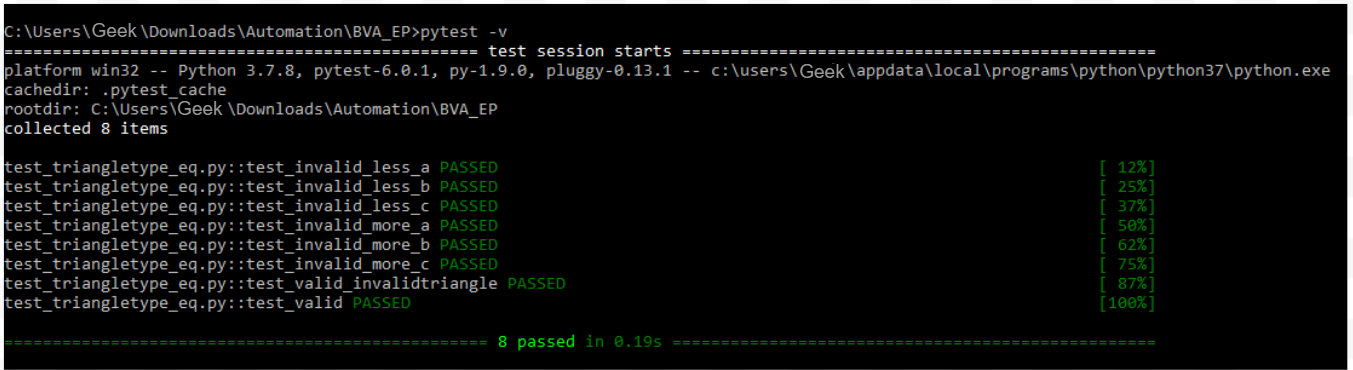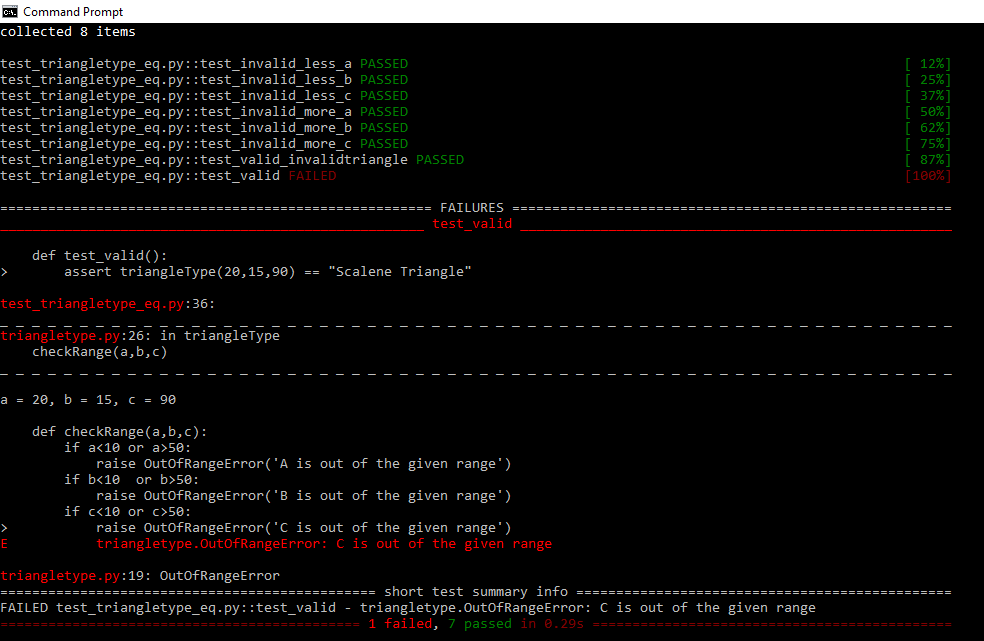# Performing Equivalence Class Testing using Pytest

• Last Updated : 10 Sep, 2020

Prerequisite – Equivalence Class Testing

To perform automated Equivalence Class Testing, we can make use of Pytest or Unittest Python libraries. In this article, we will use the Pytest library to execute test cases for a simple program.

Attention reader! Don’t stop learning now. Get hold of all the important CS Theory concepts for SDE interviews with the CS Theory Course at a student-friendly price and become industry ready.

Question :
Perform Equivalence Testing for a program that determines the type of triangle given the length of its three sides A, B, and C. The range of the length of the sides lies between 10 and 50(both inclusive).

Code in triangletype.py file :

## Python3

 `# import math`` ` `# parent class for Error``class` `Error(BaseException):``    ``pass`` ` `# child class of Error named OutOfRangeError``class` `OutOfRangeError(Error):``    ``def` `__init__(``self``, message):``        ``self``.message ``=` `message`` ` `# child class of Error named TriangleError        ``class` `TriangleError(Error):``    ``def` `__init__(``self``, message):``        ``self``.message ``=` `message`` ` `# checks if variables are in range``# if variables not in range then OutOfRangeError is raised``def` `checkRange(a, b, c):``    ``if` `a<``10` `or` `a>``50``:``        ``raise` `OutOfRangeError(``'A is out of the given range'``)``    ``if` `b<``10`  `or` `b>``50``:``        ``raise` `OutOfRangeError(``'B is out of the given range'``) ``    ``if` `c<``10` `or` `c>``50``:``        ``raise` `OutOfRangeError(``'C is out of the given range'``)`` ` `# checks if the given values of a, b, c can form a triangle``# if not, then Triangle error is raised``def` `checkTriangle(a, b, c):``    ``if` `a ``+` `b<``=` `c ``or` `b ``+` `c<``=` `a ``or` `c ``+` `a<``=` `b:``        ``raise` `TriangleError(``'Triangle cannot be formed with these sides'``)`` ` `# determines the type of triangle        ``def` `triangleType(a, b, c):``    ``checkRange(a, b, c)``    ``checkTriangle(a, b, c)``    ``# s = (a + b+c)/2``    ``# ar = math.sqrt(s*(s-a)*(s-b)*(s-c))``    ``# inradius = ar / s``    ``if``(a ``=``=` `b ``and` `b ``=``=` `c):   ``        ``return` `"Equilateral Triangle"``    ``elif``(a ``=``=` `b ``or` `a ``=``=` `c ``or` `b ``=``=` `c):``        ``return` `"Isosceles Triangle"``    ``else``:``        ``return` `"Scalene Triangle"`` ` ` ` `def` `main():``    ``try``:``        ``print``(``"Enter the sides of the triangle in range [10-50]"``)`` ` `        ``a ``=` `int``(``input``(``'Enter Side A:'``))``        ``b ``=` `int``(``input``(``'Enter Side B:'``))``        ``c ``=` `int``(``input``(``'Enter Side C:'``))``    ``except` `ValueError as v:``        ``print``(v ``+` `" Raised :Input is not an integer."``)``        ``exit(``0``)``    ``try``:``        ``checkRange(a, b, c)``    ``except` `OutOfRangeError as e:``        ``print``(``"OutOfRangeError:"` `+` `e.message)``     ` `    ``try``:``        ``checkTriangle(a, b, c)``    ``except` `TriangleError as e:``        ``print``(``'TriangleError:'` `+` `e.message)`` ` `    ``typeOfTriangle ``=` `triangleType(a, b, c)`` ` `    ``print``(``"The triangle is: "` `+` `typeOfTriangle)`` ` `if` `__name__ ``=``=` `"__main__"``:``    ``main()`

Now, we need to write test cases for the above program using the Pytest library. Each test case is written in a separate function where we use pytest.raises function to check that the given input is valid or invalid. For the above program, we create 7 invalid classes and 1 valid class. The 7 invalid classes are :

• a < 10
• a > 50
• b < 10
• b > 50
• c < 10
• c > 50
• Given values of a, b, c cannot form a triangle

The 1 valid class is :

• 10 <= a, b, c <= 50

NOTE : The function name and the test file name should always start with the word ‘test’.

Code in test_triangletype_eq.py file :

## Python3

 `import` `pytest`` ` `# importing classes and function which we use in this file``from` `triangletype ``import` `OutOfRangeError``from` `triangletype ``import` `TriangleError``from` `triangletype ``import` `triangleType`` ` `# check if a < 10``def` `test_invalid_less_a():``    ``with pytest.raises(OutOfRangeError):``        ``triangleType(``9``, ``20``, ``15``)`` ` `# check if b < 10        ``def` `test_invalid_less_b():``    ``with pytest.raises(OutOfRangeError):``        ``triangleType(``20``, ``9``, ``15``)``         ` `# check if c < 10        ``def` `test_invalid_less_c():``    ``with pytest.raises(OutOfRangeError):``        ``triangleType(``20``, ``15``, ``9``)`` ` ` ` `# check if a > 50``def` `test_invalid_more_a():``    ``with pytest.raises(OutOfRangeError):``        ``triangleType(``51``, ``30``, ``45``)``         ` `# check if b > 50        ``def` `test_invalid_more_b():``    ``with pytest.raises(OutOfRangeError):``        ``triangleType(``30``, ``51``, ``45``)``         ` `# check if c > 50        ``def` `test_invalid_more_c():``    ``with pytest.raises(OutOfRangeError):``        ``triangleType(``30``, ``45``, ``51``)`` ` `# check if a, b, c can form a triangle or not        ``def` `test_valid_invalidtriangle():``    ``with pytest.raises(TriangleError):``        ``triangleType(``20``, ``15``, ``40``)`` ` `# valid class - determines type of triangle        ``def` `test_valid():``    ``assert` `triangleType(``20``, ``15``, ``10``) ``=``=` `"Scalene Triangle"`

To execute the above test cases, create two separate files triangletype.py and test_triangletype_eq.py in a single folder. To execute write the following command :

```pytest
```

OR

```pytest -v
```

pytest -v shows the verbose output.

The output is shown below :As we can see in the above output, all the 8 test cases passed. However, if we edit a test case, for example, if we change the value of c variable to 90 in the test_valid() test case in test_triangletype_eq.py file, then the test case fails :My Personal Notes arrow_drop_up